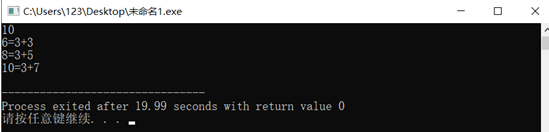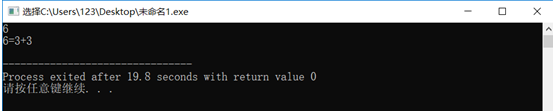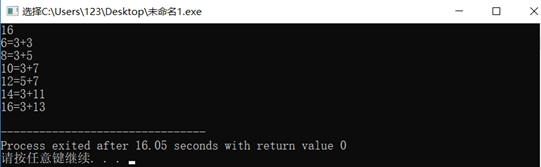# 检验“哥德巴赫”猜想

1）  检验6－n（n>=6）之内的偶数

2）  每个偶数写成两个整数之和的形式 例：6=3+3 8=3+5  10=3+7 等,

1、数据结构的设计（写出构造的数据类型，即自己定义的结构体)

2、算法分析(算法用流程图或自然语言描述)

6~n之内每个偶数所分成的两个数，再调用布尔函数判断那两个数是否同时为素数，若同时为素数，则输出每个偶数写成两个整数和的形式；若两个数不全是素数，则跳出两个for循环输出Wrong.

1、源程序（见附录）

2、测试结果截图 写出测试程序的数据，至少写三组数据，并把每组测试结果截图）

（1）第一组测试数据及结果截图

N=6（2）第一组测试数据及结果截图

N=10（3）第一组测试数据及结果截图

N=161.由于本程序先写的布尔函数构造的判断素数的函数，为了结构简明，将所有的变量设成全局变量

2.在不符合条件时，输出Wrong时应跳出两个for循环，否则会输出多个Wrong(本题并不会出现输出Wrong的情况)

3.调用函数判断两个数是否同时为素数，采用&&，简明

#include<stdio.h>
#include <stdbool.h>
int n,a,k,i,j;
bool su(int n)//构造判断是否为素数的布尔函数；
{
for(k=2;k<n;k++)
if(n%k==0)break;
if(k<n)
return false;
else
return true;
}
int main()
{
scanf("%d",&n);
for(i=6;i<=n;i=i+2)
{
for(j=3;j<n;j++)
{
a=i-j;
if(su(a)&&su(j))
{
if(j<=a)
printf("%d=%d+%d\n",i,j,a);
break;//在符合条件的情况下，break保证只输出一种情况；
}

}
}
if(!su(a)&&su(j))//跳出两个for循环，防止输出多个Wrong ;
{
printf("Wrong!");
}
return 0;
}

posted @ 2021-05-31 09:21  烤山药护体  阅读(24)  评论(0编辑  收藏  举报